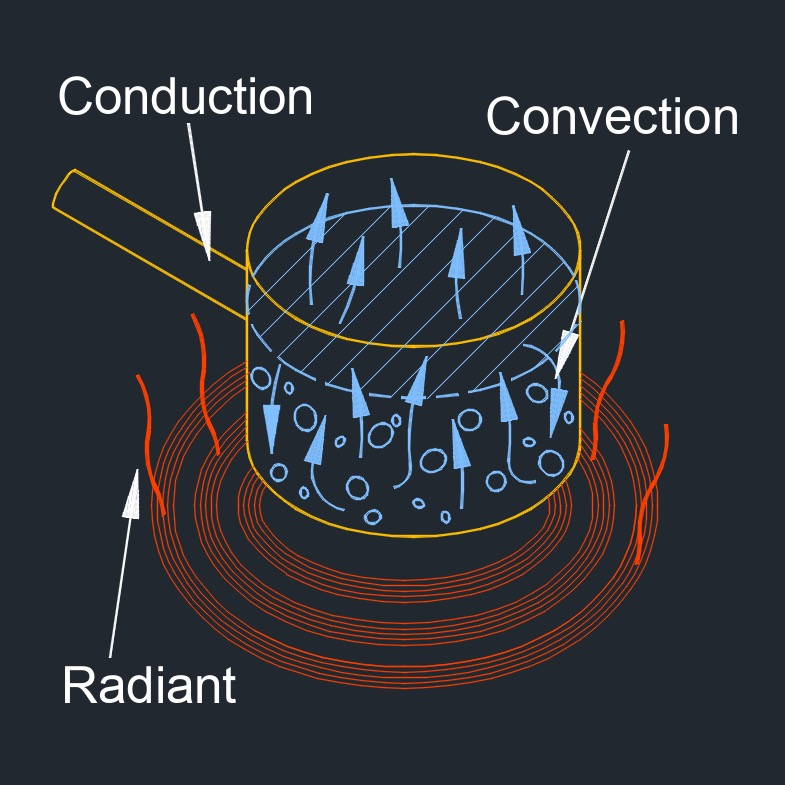# Heat of Vaporization

on . Posted in ThermodynamicsHeat of vaporization, abbreviated as $$H_v$$, is the amount of heat needed to be consumed to vaporize a specific amount of liquid at a constant temperature.

## Heat of vaporization Formula

$$\large{ H_v = \frac{ q }{ m } }$$
Symbol English Metric
$$\large{ H_v }$$ = heat of vaporization $$\large{\frac{Btu}{lbm}}$$   $$\large{\frac{J}{kg}}$$
$$\large{ q }$$ = heat $$\large{\frac{Btu}{lbm}}$$ $$\large{\frac{J}{kg}}$$
$$\large{ m }$$ = mass of substance $$lbm$$ $$kg$$